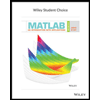# Assume that a procedure yields a binomial distribution with n=1222n=1222 trials and the probability of success for one trial is p=38%p=38%.Find the mean for this binomial distribution.(Round answer to one decimal place.)μ=μ= Find the standard deviation for this distribution.(Round answer to two decimal places.)σ=σ= Use the range rule of thumb to find the minimum usual value μ–2σ and the maximum usual value μ+2σ.Enter answer as an interval using square-brackets only with whole numbers.usual values =

Question

Assume that a procedure yields a binomial distribution with n=1222n=1222 trials and the probability of success for one trial is p=38%p=38%.

Find the mean for this binomial distribution.
(Round answer to one decimal place.)
μ=μ=

Find the standard deviation for this distribution.
(Round answer to two decimal places.)
σ=σ=

Use the range rule of thumb to find the minimum usual value μ–2σ and the maximum usual value μ+2σ.
Enter answer as an interval using square-brackets only with whole numbers.
usual values =

Expert Solution

### Want to see the full answer?

Check out a sample Q&A hereStudents who’ve seen this question also like:MATLAB: An Introduction with Applications
6th Edition
ISBN: 9781119256830
Author: Amos Gilat
Publisher: John Wiley & Sons Inc